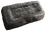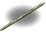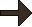# Template:Craft use table

This template is identical in almost every way to `{{Grid/Crafting Table}}`, except it does not categorize the page using it as a "Craftable item"

## Usage

### Basic usage

```{{Craft use table
|A1=  |B1=  |C1=  |D1=  |E1=
|A2=  |B2=  |C2=  |D2=  |E2=
|A3=  |B3=  |C3=  |D3=  |E3=
|A4=  |B4=  |C4=  |D4=  |E4=
|A5=  |B5=  |C5=  |D5=  |E5=
|Output=
}}```

### Examples

```{{Craft use table
|A1=  |B1=  |C1=  |D1= IngotIron  |E1=
|A2=  |B2=  |C2=  |D2= Stick |E2= IngotIron
|A3=  |B3=  |C3= Stick  |D3=  |E3=
|A4=  |B4= Stick  |C4=  |D4=  |E4=
|A5=  |B5=  |C5=  |D5=  |E5=
|Output= Fireaxe,1
|time= 10 min
}}```
producesCrafting time: 10 min